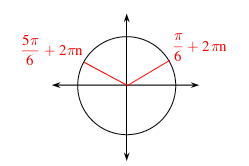### Home > CCA2 > Chapter Ch12 > Lesson 12.1.3 > Problem12-53

12-53.

 For each of the following problems, find all of the solutions without using a calculator. Use a graph or unit circle to support youranswers. Then predict the solution that your calculator will give and use your calculator to check your prediction.
1. $4\sin(x)+2=0$

Subtract 2 from both sides and divide by $4$ to solve for $\sin(x)$.

$\sin(x)=\frac{1}{2}$

On a unit circle, find the two different times $\sin(x)=\frac{1}{2}$. To give all of the solutions, remember that every time you go around the circle, that value occurs again. Since there are $2π$ radians in a circle, add $2πn$ to each solution.1. $2\cos(x)=\sqrt{3}$

Use the same method as part (a).

$= \frac{\pi}{6}+2{\pi}n,\;\frac{11\pi}{6}+2{\pi}n$

1. tan $(x) + 1 = 0$

Use the same method as part (a). How is the tangent function different from the sine function?

1. $4$ cos2$(x)- 4 = 0$

$x=\pi n$